## 1. Write the equation of the line for the points (2,-1) and (-4,11).

Question

1. Write the equation of the line for the points (2,-1) and (-4,11).

in progress 0
2 weeks 2021-09-02T05:51:50+00:00 1 Answers 0 views 0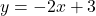Step-by-step explanation:

Slope-intercept form of an equation is written as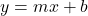, where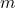is the slope and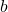is the y-intercept.

The slope of a line that passes through the points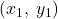and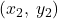is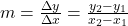. Using the coordinates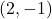and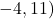as given in the problem, we have slope of this line to be: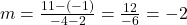.

Now using this slope we’ve found and any point the line passes through, we can find the y-intercept of this equation: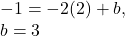Therefore, the equation of this line in slope-intercept form is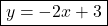.